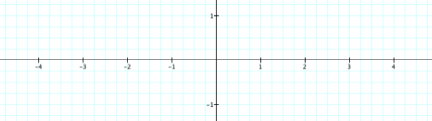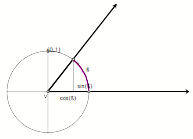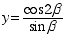Write your answers to these questions while clearly keeping in mind the mantra, "Variables vary; go slow!"

1. Jimmy said, "sin( ) has a period of 2", which means that it repeats itself whenever whatever is in the parentheses varies by 2π. So, sin(5+3x) repeats itself whenever 5+3x varies by 2π."
1. What does Jimmy mean?

1. Use Jimmy's insight to sketch a graph of these functions and to explain their behaviors:

y = cos(7x), -π <= x <= π?y = cos(1/x), -π <= x <= π?1.Use an image of the unit circle to:
1. Sketch a graph of, 0 <= β <= 2π.

1. Explain why the graph of, 0 <= β <= 2π, behaves as it does.